# How to Subtract Two Matrices

This article was written and distributed with the intention of describing the process by which two matrices are subtracted together. So without further ado, let's get right down to defining what exactly we mean when we say "matrix."

## What is a matrix?

A matrix is the term used to refer to a collection of numbers that are laid out in the format of rows and columns. For example: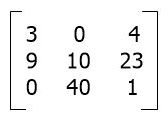The matrix that was just shown has three rows, and each row has three columns. As a result, there are a total of nine different elements or numbers that can be chosen from within this matrix. Let's get down to business and talk about the meat of the issue, which is the subtraction of two matrices or the process of subtracting matrices together.

## Matrix subtraction

Let me explain the one necessary condition that must be met before we can proceed with the matrix subtraction. This condition is required in order for the matrix subtraction to take place. In subtraction, the requirement is that both matrices have an identical number of rows and columns.

Now is the time to set an example. Let's suppose that there are two given matrices, matrix A and matrix B. The value of Matrix A is:

```5  6
7  8```

And the value of Matrix B is:

```1  2
3  4```

Then its subtraction is as follows: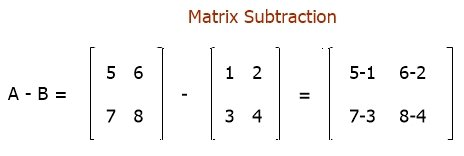So we have another matrix, matrix C. The value of matrix C is:

```4  4
4  4```

This is the subtraction of two given matrices, A and B.

### Further Explanation of Matrix Subtraction

As already explained, for the matrix subtraction, there should be an equal number of rows and columns. A null matrix is produced when a matrix is subtracted from itself, or when A-A = O.

Matrix subtraction is the addition of the negative of one matrix to another, i.e., A - B = A + (-B). The relevant elements are also subtracted in order to subtract matrices.

Given A and B matrices as follows, find A - B. The matrix A =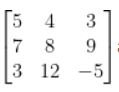And the Matrix B =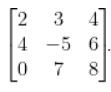In this way, the answer can be found by subtracting the matching elements from matrices A and B.So the value of Matrix A - Matrix B will be: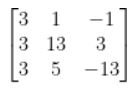#### Programs created for matrix subtraction

« Previous Topic Next Topic »